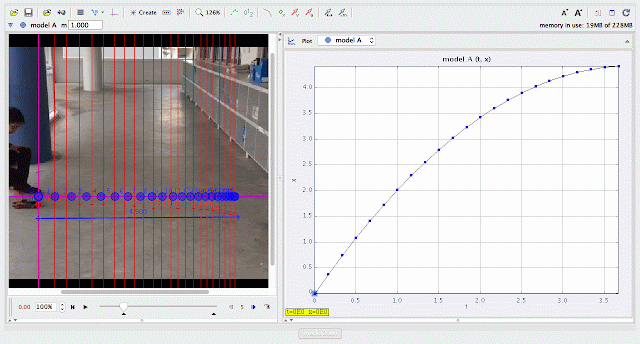{source}
<?phprequire_once JPATH_SITE.'/TTcustom/TT_contentparser.php';//include 'TT_contentparser.php';$$parameters = array( "topicname" => "02_newtonianmechanics_2kinematics", //e.g. 02_newtonianmechanics_2kinematics"modelname" => "andytoycar2_5"); //e.g. airpuckconstantvelocity.trzecho generateSimHTML($$parameters, "TRZ");
?>
{/source}

### ICT Connection Lessonusing Tracker Model Builder to create theoretic graphs for simplifying Physics concepts. concept illustrated is uniform deceleration displacement graphusing Tracker Model Builder to create theoretic graphs for simplifying Physics concepts. constant deceleration is illustrated through straight line of velocity versus time graphusing Tracker Model Builder to create theoretic graphs for simplifying Physics concepts. constant deceleration is illustrated through horizontal straight line of acceleration versus time graph

end faq

Rating 5.00 (1 Vote)